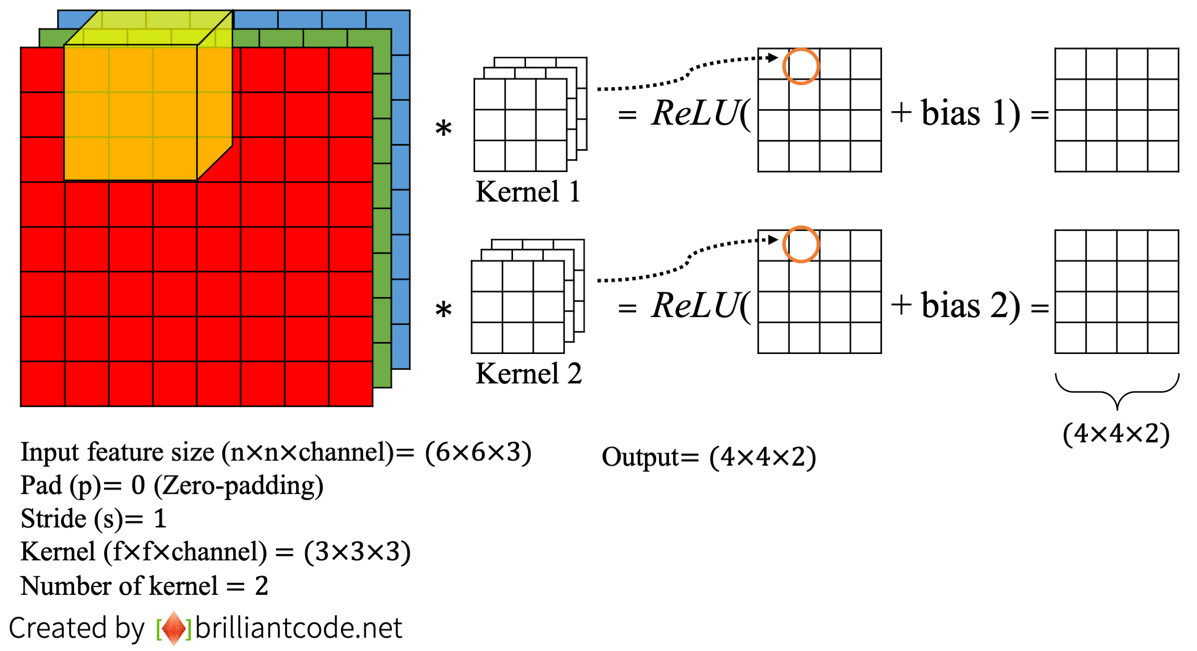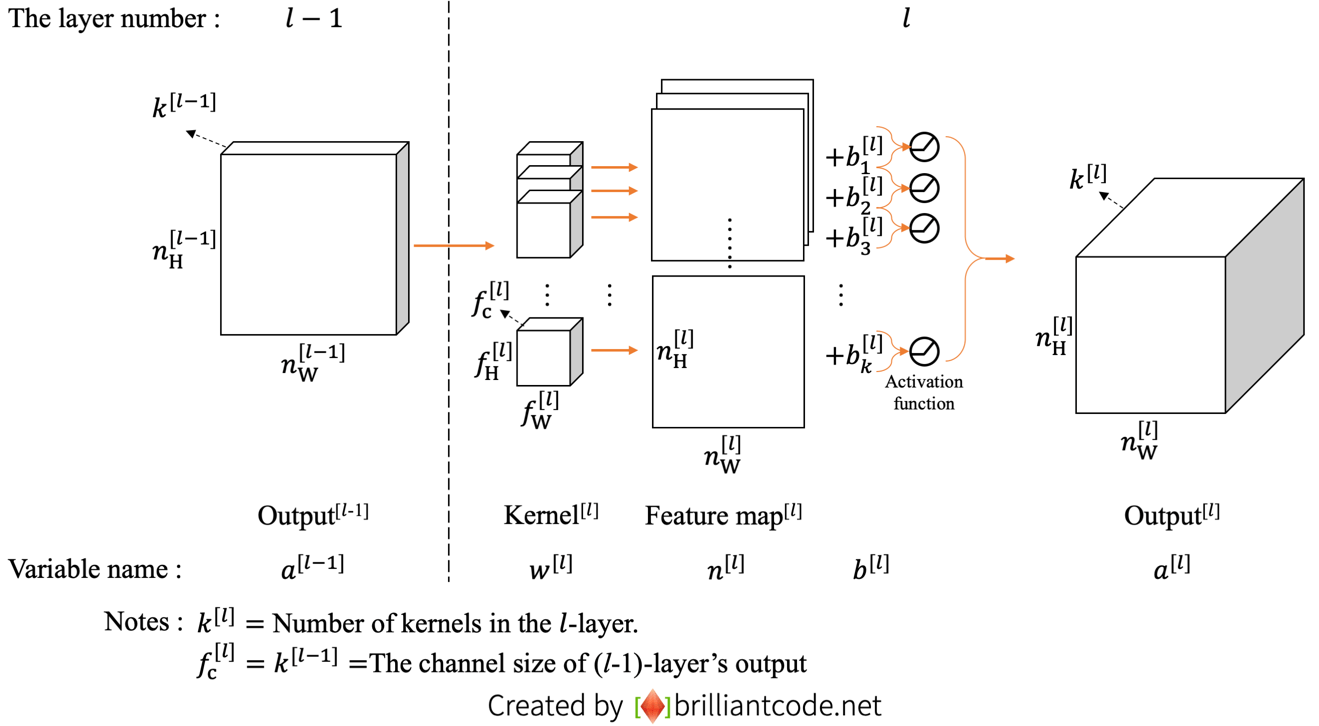# Convolutional Neural Networks(CNN) #3 計算參數量

CNN（Convolutional Neural Networks）對電腦視覺領域來說可以說是非常有效，而且參數計算方式對新手來說卻是很複雜的，所以我們先用一個簡單的單層CNN說明CNN運算流程，之後再來看參數量計算與完整的CNN架構會更清楚易懂。

bias 1、bias 2皆為純量實數圖（1）：多卷積核的單層CNN運算流程

 符號 說明 上標$^{[l]}$ 表示第$l$層的變數 $a$ 代表每一層的類神經網路輸出 $k$ Kernel數量 $w$ 表示卷積核 $n$ 表示完成卷積運算的Feature map $b$ 表示偏差值（Bias） $n_{W}$ 完成卷積運算Feature map的寬度 $n_{H}$ 完成卷積運算Feature map的高度 $f_{W}$ 卷積核的寬度 $f_{H}$ 卷積核的高度 $f_{c}$ 卷積核的深度（Channel數）Ans：答案就是$(3\times3\times3+1)\times10=280$（記得要算Bias喔！）

Reference